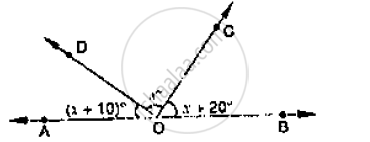# In the Given Below Fig, Find X. Further Find ∠Boc, ∠Cod and ∠Aod . - Mathematics

In the given below Fig, find x. Further find ∠BOC, ∠COD and ∠AOD .#### Solution

Since ∠AOD and ∠BOD are form a line pair

∠AOD + ∠BOD = 180°

∠AOD + ∠COD + ∠BOC = 180°

Given that

∠AOD = ( x +10)°, ∠COD = x°, ∠BOC = ( x + 20)°

( x +10)° + x° + ( x + 20)° = 180°

3x + 30° = 180°

⇒ 3x = 180° - 30°

⇒ 3x = 150°

⇒ x = 50°

∴∠AOD = x +10°

= 50° +10° = 60°

∠COD = x° = 50°

∠BOC = x + 20° = 50 + 20 = 70°

Concept: Pairs of Angles
Is there an error in this question or solution?

#### APPEARS IN

RD Sharma Mathematics for Class 9
Chapter 10 Lines and Angles
Exercise 10.2 | Q 3 | Page 14